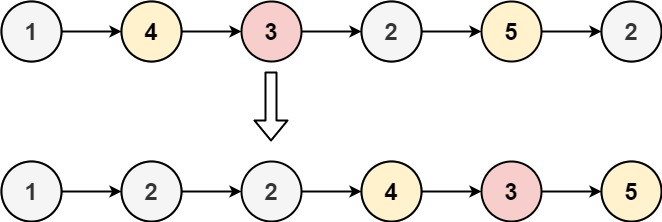| English | 简体中文 |

# 86. Partition List

## Description

Given the head of a linked list and a value x, partition it such that all nodes less than x come before nodes greater than or equal to x.

You should preserve the original relative order of the nodes in each of the two partitions.

Example 1:Input: head = [1,4,3,2,5,2], x = 3
Output: [1,2,2,4,3,5]


Example 2:

Input: head = [2,1], x = 2
Output: [1,2]


Constraints:

• The number of nodes in the list is in the range [0, 200].
• -100 <= Node.val <= 100
• -200 <= x <= 200